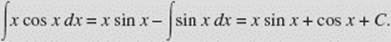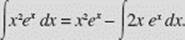﻿ ﻿INTEGRATION BY PARTS - Antidifferentiation - Calculus AB and Calculus BC

## CHAPTER 5 Antidifferentiation

### D. INTEGRATION BY PARTS

Parts Formula

The Parts Formula stems from the equation for the derivative of a product:or, or more conveniently d(uv) = u dv + v du.

Hence, u dv = d(uv) − v du and integrating gives usorthe Parts Formula. Success in using this important technique depends on being able to separate a given integral into parts u and dv so that (a) dv can be integrated, and (b)du is no more difficult to calculate than the original integral.

EXAMPLE 43

FindSOLUTION: We let u = x and dv = cos x dx. Then du = dx and v = sin x. Thus, the Parts Formula yieldsEXAMPLE 44

FindSOLUTION: We let u = x2 and dv = ex dx. Then du = 2x dx and v = ex, soWe use the Parts Formula again, this time letting u = x and dv = ex dx so that du = dx and v = ex. Thus,EXAMPLE 45

Find I =SOLUTION: To integrate, we can let u = ex and dv = cos x dx; then du = ex dx, v = sin x. Thus,To evaluate the integral on the right, again we let u = ex, dv = sin x dx, so that du = ex dx and v = − cos x. Then,EXAMPLE 46

FindSOLUTION: We let u = ln x and dv = x4 dx. Then,andThus,THE TIC-TAC-TOE METHOD. 1

This method of integrating is extremely useful when repeated integration by parts is necessary. To integratewe construct a table as follows:Here the column at the left contains the successive derivatives of u(x). The column at the right contains the successive antiderivatives of v(x) (always with C = 0); that is, v1 (x) is the antiderivative of v(x), v2 (x) is the antiderivative of v1 (x), and so on. The diagonal arrows join the pairs of factors whose products form the successive terms of the desired integral; above each arrow is the sign of that term. By the tic-tac-toe method,EXAMPLE 47

To integratecos x dx by the tic-tac-toe method, we let u(x) = x4 and v(x) = cos x, and get the following table:The method yieldsWith the ordinary method we would have had to apply the Parts Formula four times to perform this integration.

1 This method was described by K. W. Folley in Vol. 54 (1947) of the American Mathematical Monthly and was referred to in the movie Stand and Deliver.

﻿